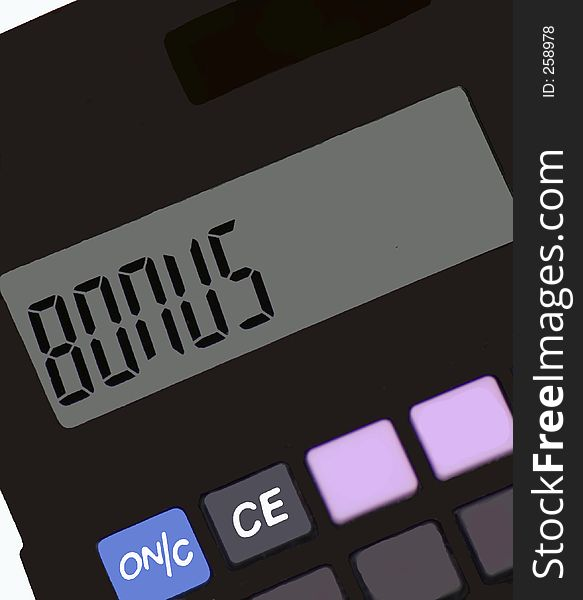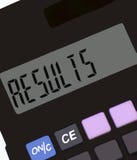Calculator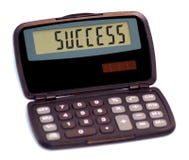Calculator II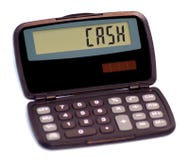Calculator II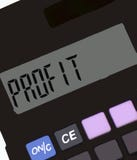Calculator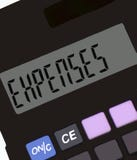Calculator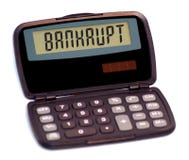Calculator II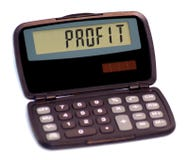Calculator II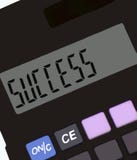CalculatorDismissal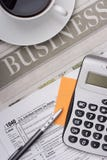Form For Tax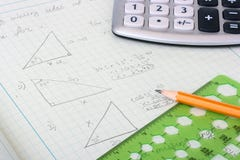Geometrical CalculationsYoung Bearded Man Counts On A CalculatorCalculator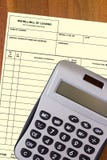Calculator With A Blank Waybill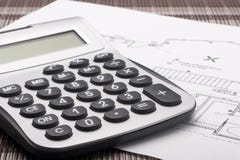Calculator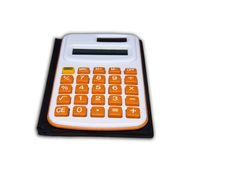CalculatorBusinessman Showing Calculator With 1000+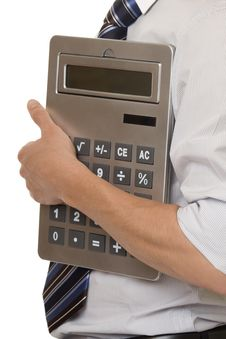Businessman With Gigantic Pocket Calculator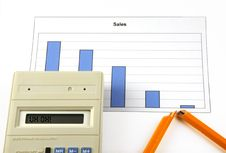Calculator With Text Uh Oh!, Graph And Broken PenMan With CalculatorCalculator With Hand